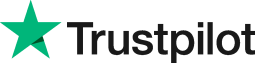How to calculate age from a date of birth in Excel
• Home
• Courses
• Promotions
• Schedule
• Formats
• Our Clients

Forum home » Topics » How to calculate age from a date of birth in Excel

# How to calculate age from a date of birth in Excel

There are many calculations that can be done in Excel and some of them involve working with dates. Excel sees all dates as numbers and when you subtract one date from another the result will be a number.

Here are two methods of calculating age from a date of birth in Excel: a formula method using three cells, and the DATEDIF function.

1 How to calculate age using a formula.

To calculate your age from your date of birth in Excel requires using three cells.

In the first cell, let's say A1, type the following formula:

=TODAY() press enter

In cell A2, enter your birth date e.g. 12/03/1982

In cell A3, type the following formula:

=(A1-A2)/365.25 press enter

Doing this will calculate your age as required. The result usually contains a lot of decimal places and to reduce these you can create the formula as follows:

=INT((A1-A2)/365.25)

Combining functions in formulas provides a dynamic way of manipulating data and is commonly used to some quite complex calculations.

2 How to calculate age from a date of birth using the DATEDIF function

Another way to calculate age is with the DATEDIF function.
=DATEDIF(Date1, Date2, Interval)

where Date1 is the birthdate, Date2 is today's date and Interval is the interval type ("y" for years "m" for months and "ym" for months in the same year).

This formula displays age in years for a date in cell A1:
=DATEDIF(A1,TODAY(),"y")

This formula displays age in years and months:
=DATEDIF(A1,TODAY(),"y")&" years "&DATEDIF(A1,TODAY(),"ym")& " months"

# Related forum posts:

## Getting Age from Date of Birth Formula

I have exported some data to excel, which has the candidate date fo birth, I know there is a formula within excel allowing it calculate the age for all those candidates....Does anyone know how to do it? Fingers crossed Thanks in advance.....a Swift response would be appreciated.

## How to calculate age on a particular date

How does one calculate a list of peoples' ages on a particular date easily?

# Related articles

## How Are Dates Stored In Excel?

Have you ever wondered how a date is actually stored in Excel? This article describes how Excel stores dates and covers an example of how this can be used in calculations.

## Find Out Your Age In Days With Excel

Would you like to know how many days you've been alive? This simple formula in Excel allows you to find out. By using the same simple rules, you could take the process further and find out how many hours, or even minutes you have been here.

## Sorting Birthdates Into Their Respective Months In Excel

Shady deeds are afoot at Dodd Gee Enterprises. An email is being sent out to their entire customer base with a free horoscope reading that is intended to persuade the customer to buy a targeted product. In order to do this, the dates of birth of the entire customer list need to be separated into months. This article explains how this is done.

## Using Excel 2007/2010's Formula Checking Features

Find out how Excel can help you determine visually which cells are used in a formula, and learn how to use alerts and formula auditing for further investigation.

## The Secret Formula To Creating Correct Calculations In Excel

Did you know that remembering and understanding a basic maths calculation could save you time and money when using Excel? Find out if you need to go back to the classroom before applying correct formula to your worksheets.

 Welcome. Please choose your application (eg. Excel) and then post your question. Our Microsoft Qualified trainers will then respond within 24 hours (working days). Frequently Asked Questions What does 'Resolved' mean? Any suggestions, questions or comments? Please post in the Improve the forum thread.TrustScore 4.9 / 5       1244 reviews